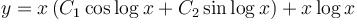Courses

# Differential Equation MCQ Level - 1

## 10 Questions MCQ Test Topic wise Tests for IIT JAM Physics | Differential Equation MCQ Level - 1

Description
This mock test of Differential Equation MCQ Level - 1 for Physics helps you for every Physics entrance exam. This contains 10 Multiple Choice Questions for Physics Differential Equation MCQ Level - 1 (mcq) to study with solutions a complete question bank. The solved questions answers in this Differential Equation MCQ Level - 1 quiz give you a good mix of easy questions and tough questions. Physics students definitely take this Differential Equation MCQ Level - 1 exercise for a better result in the exam. You can find other Differential Equation MCQ Level - 1 extra questions, long questions & short questions for Physics on EduRev as well by searching above.
QUESTION: 1

### The integrating factor of the differential equation,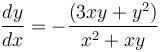would be :

Solution:

The given differential equation is homogeneous
Consider,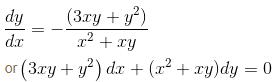Comparing it with the equation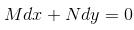We have,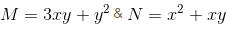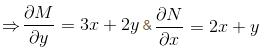Also, the equation is homogeneous &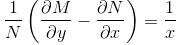∴  The integrating factor would be given by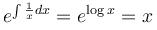QUESTION: 2

### The curve r = a(secθ + tanθ) is orthogonal to :

Solution:

Given,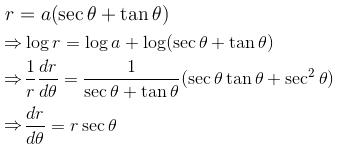Replacing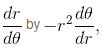to get the orthogonal curve,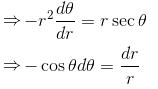Integrating both sides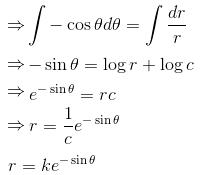The correct answer is: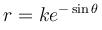QUESTION: 3

### Solution to the differential equation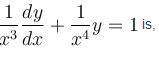Solution: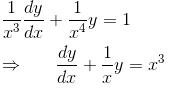which is a linear equation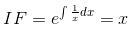The required solution will be given by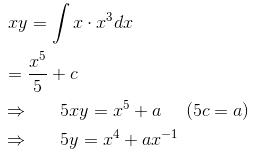5y = x4 + ax–1

QUESTION: 4

What would be the order and degree of the given differential equation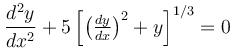Solution:

Consider the differential equation,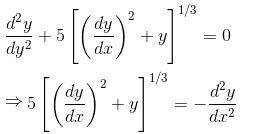Cubing both sides,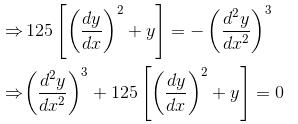∴  according to definition, degree of the above differential equation is 3 and order is 2.

The correct answer is: Order–2 & Degree–3

QUESTION: 5

The general solution of the differential equation,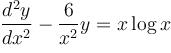will be given by.

Solution:

We havePutting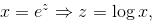the above equations becomes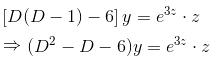Auxiliary equation :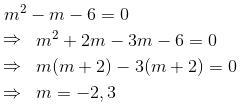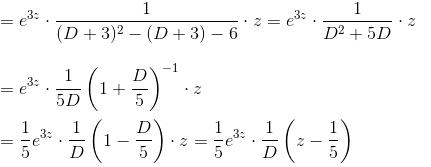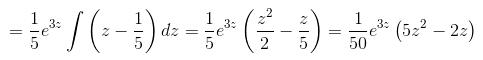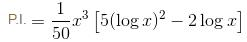Hence,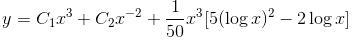The correct answer is: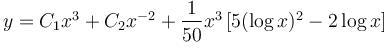QUESTION: 6

The general solution of the differential equation,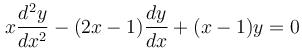will be given by :

Solution:

Since y = ex is an integral, we take y = ve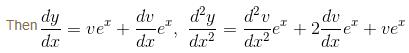The given equation becomes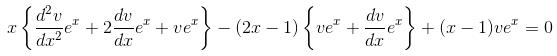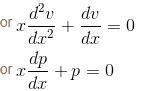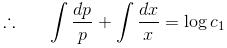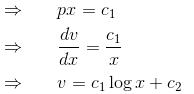Hence y = vex = (c1 log x + c2)ex is the complete solution.

The correct answer is: (C1 log x + C2)ex

QUESTION: 7

The particular integral of the differential equation,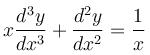will be

Solution:

Multiplying the given equation by x2 we obtain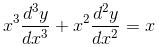Then the equation, on putting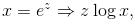becomes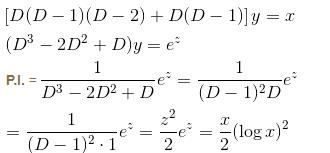The correct answer is: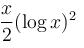QUESTION: 8

The solution of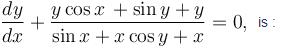Solution: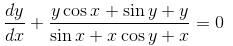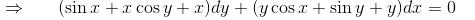Comparing it with the equation,
Mdx + Ndy = 0
We have,
M = y cos x + sin y + y    &     N = sin x + x cos y + x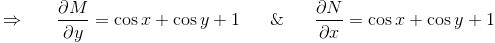Hence, the given differential equation is exact.
∴  The required solution will be given by,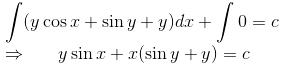The correct answer is: y sin x + (sin y + yx = c

QUESTION: 9

The differential equation,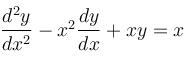can be reduced into which of the following linear equation?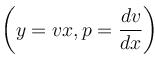Solution:

We have P + Qx = –x2 + x2 = 0 and so y x is an integral.

Let y = vx so that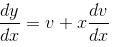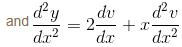Now the given equation reduces to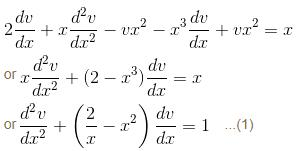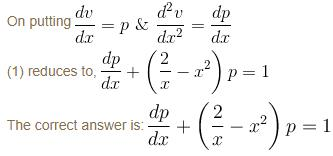QUESTION: 10

Which of the following function form the solution of the differential equation, [x2D2 – xD + 2]y = log x?

Solution:

On putting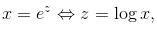the given equation becomes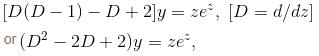The auxiliary equation is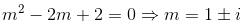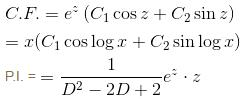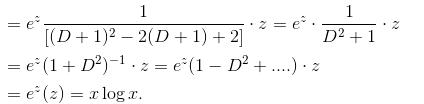Hence,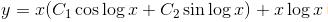is the solution:

The correct answer is: